# Talk:06-240/Homework Assignment 4

Jump to: navigation, search

## Divisibility by Prime Number

Pls correct me if I were wrong. The operation of cut away the unit digit is a distraction. If we consider the unit digit, the operation basically is a deduction of a number, and that number is divisible by 7. The whole operation is shown as follow:

 86415 105 105/7=21 ---- 8631 21 21/7=3 --- 861 21 21/7=3 -- 84 84 84/7=12 - 0 0/7=0

Since it is an operation of series subtraction by multiples of 7, therefore the number we started from is divisible by 7 iff the resulting number is divisible by 7.

Moreover, there is a relationship between the unit digit, 2, and 7. The unit digit multiple by 21(7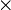$\times$ 3) is equal to the combination of the unit digit with its 2-time as the tenth/hundredth digit.

Unit digit,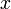$x$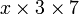$x \times 3 \times 7$
0 0
1 21
2 42
3 63
4 84
5 105
6 126
7 147
8 168
9 189

From the table above, I've induced the criterion for divisibility by 17 that is similar operation but the unit digit multiplies by 5 instead of 2. For divisibility by 13, the unit digit multiple by 9. Alright, I think it will be more fun if it's explained by other people. Wongpak 09:38, 5 October 2006 (EDT)

## Excellent!

--Drorbn 12:09, 5 October 2006 (EDT)

I found the problem solved three different ways at Jim Loy's divisibility page He mentions the problem as stated can be found in The Dictionary of Curious and Interesting Numbers by David Wells.

I prefer his second method. Beginning from the right most digit, multiply corresponding digits by the following sequence of coefficients 1, 3, 2, 6, 4, 5. For larger numbers sequence repeats. Alternatively the sequence 1, 3, 2, -1, -3, -2 could be used.

Using the given example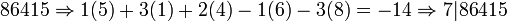$86415\Rightarrow 1(5)+3(1)+2(4)-1(6)-3(8)=-14\Rightarrow 7|86415$

Coefficients can be determined by taking multiplying the previous coefficient by 10 and take the modulus of this new number.

For n=17, sequence begins with 1 multiply by 10 then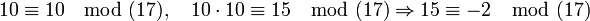$10\equiv 10 \mod(17),\quad 10\cdot 10\equiv 15\mod(17) \Rightarrow 15\equiv -2\mod(17)$

So for n=17, sequence is: 1, 10, 15, 14, 4, 6, 9, 5, 16, 7, 2, 3, 13, 11, 8, 12

or alternatively: 1, -7, -2, -3, 4, 6, -8, 5, -1, 7, 2, 3, -4, -6, 8, -5

## Nice, but...

but for each divisibility you have to memorize (or calculate again and again) a whole sequence of numbers, rather than just one. --Drorbn 10:30, 10 October 2006 (EDT)